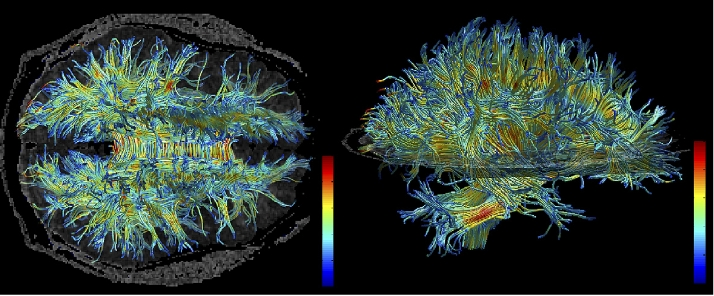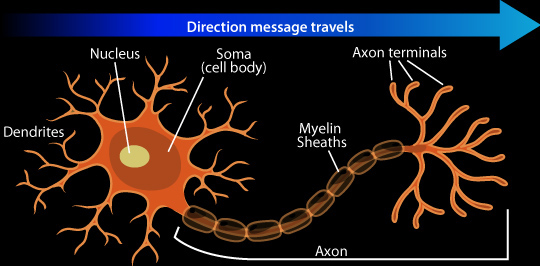## Definition

### What is a neural network?

a computer system modelled on the human brain and nervous system

### What is brain?

With no doubt it is a giant nebula (1014) of nervous cells interconnected by 1.7 * 108 meters of bound tissue.Figure 1: Interconnections

### What is a neuron?Figure 2: Neuron anatomy

Nerve Cell: Dendrites receive messages from other neurons. The message then moves through the axon to the other end of the neuron, then to the tips of the axon and then into the space between neurons. From there the message can move to the next neuron.

## Implementation

### Inlet

class Inlet(object):
"""Input signal into dendron"""
def __init__(self):
pass
def modulate(self, signal):
self.signal = signal


### Dendron

class Dendron(object):
"""Convey signal to neuron"""
def __init__(self, neuron, inlet, weight=0.1):
self.neuron = neuron
self.weight = weight
self.inlet = inlet


### Soma

class Neuron(object):
"""Processing module itself"""
def __init__(self, df=lambda x: x):  # sigmoidal
self.df = df
self.dendrons = []
self.output = 0
def calculate_output(self):
for dendron in self.dendrons:
self.output += self.df(
dendron.weight *
dendron.inlet.signal)


### Neural Network

class NeuralNetwork(object):
"""Ties all classes above together"""
def __init__(self, swing):
"""Creates network with swing number of inlets and equal number of
neurons interconnected by dendrons

"""
self.network_swing = swing
self.inlets = []
self.neurons = []
self.dendrons = []
for i in range(swing):
self.inlets.append(Inlet())
self.neurons.append(Neuron())
for j in range(swing):
self.dendrons.append(
Dendron(self.neurons[-1],
self.inlets[-1]))
self.neurons[-1].dendrons.append(self.dendrons[-1])
def eval_input(self, input_array):
for i in range(self.network_swing):
self.inlets[i].modulate(input_array[i])
for i in self.neurons:
i.calculate_output()
def update_weights(self, weights_array):
for i in range(self.network_swing ** 2):
self.dendrons[i].weight = weights_array[i]


### Test suite

#### Network initialization

NN = NeuralNetwork(2)
NN.update_weights([0.1, 0.2, 0.3, 0.4])
for i in NN.dendrons:
print(i.weight)


>>> ... ... 0.1
0.2
0.3
0.4



#### Input handling

NN.eval_input([1, 1])
for i in NN.neurons:
print(i.output)


... ... 0.30000000000000004
0.7